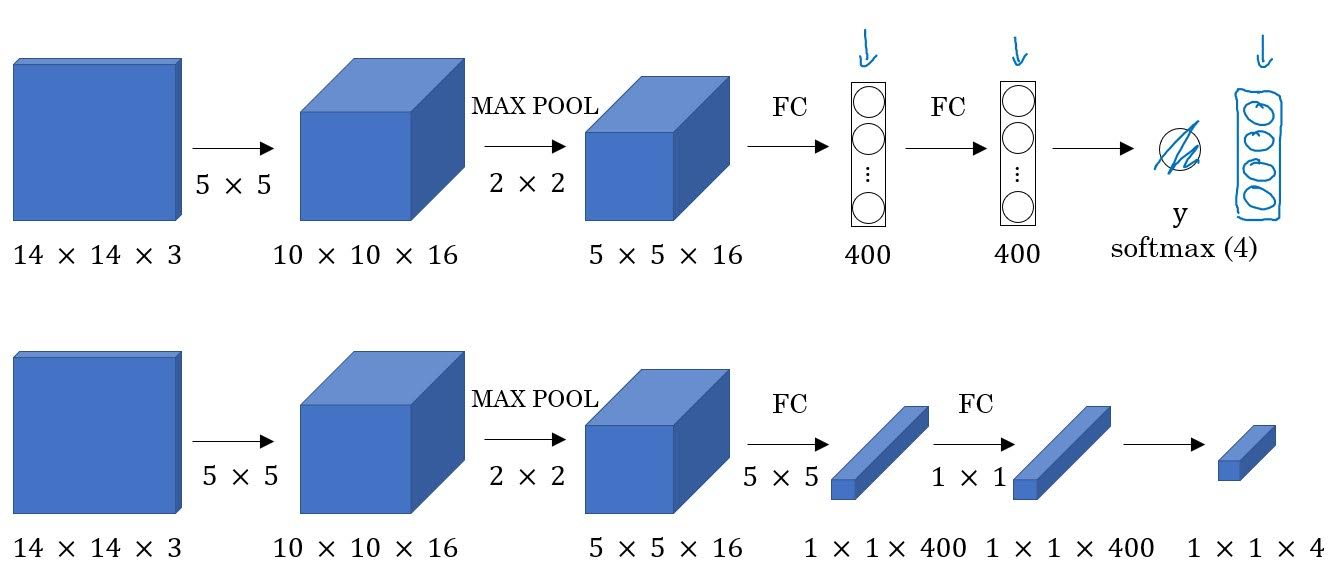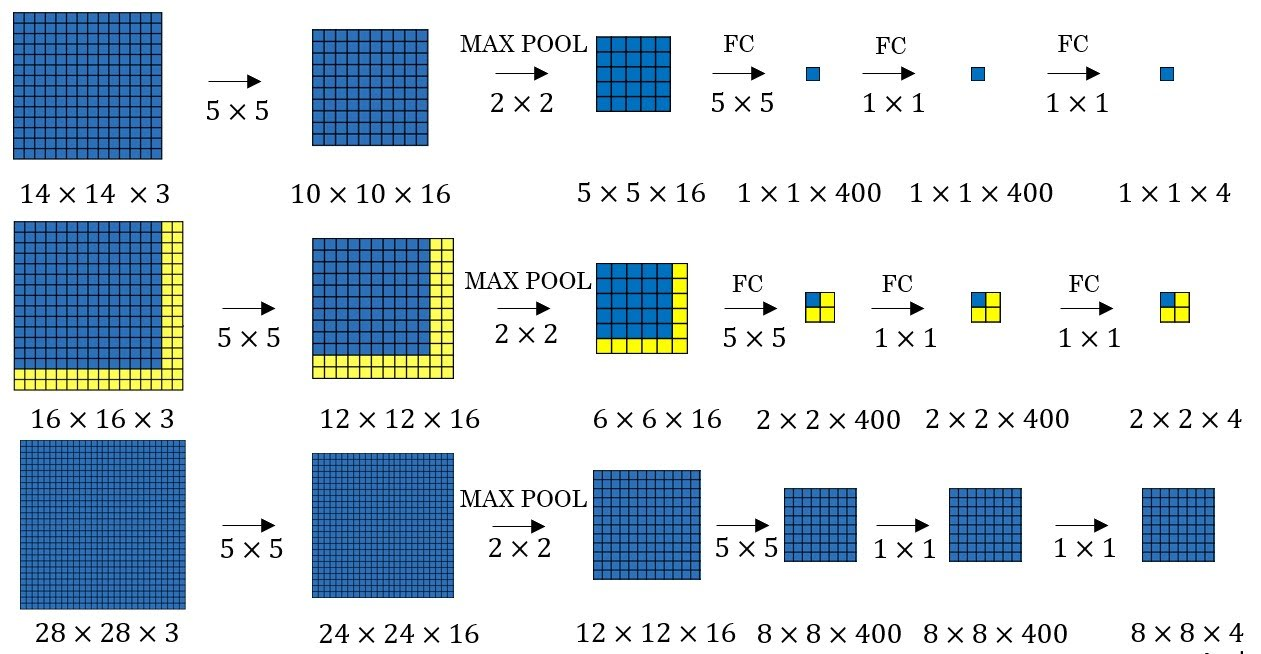Categories:

Updated:

# Sliding Windows Algorithm

1. train a image classifier.
2. Put a ‘window’ on an image and run that part of the image through trained classifier. Slide the window and do it again.
3. Increase the window size and repeat 2.# Computational Cost

This algorithm is computationally expensive, since you need to run your classifier many many times.

If you increase the stride, or try few window sizes, computational cost will decrease but its performance will decrease too.

# Convolutional Implementation

There is a way to save cost.

## Turning FC Layers into Convolutional LayersThere are two ways to make 5x5x16 volume into a vector of 400 elements, as described above.

First, you can flatten the 5x5x16 volume into a 1-dimensional vector of 400 elements, then use fully-connected(dense) layer to output units.

Second, you can apply convolution with 400 5x5x16 filters.

Mathematically these two methods are the same.

## Convolutional Implementation of Sliding WindowsNote that the above units are actually 3-dimensional, but for the sake of simplicity drawn in 2-dimensional.

First row of the above image is convolutional network that classifies 14x14x3 sized image. So the size of the window is 14x14.

In the second row, same convolution network is applied to 16x16x3 image where we want to detect object. Top-left value of the final output is actually the predicted label of the window applied to the top-left part of the input image. These are represented in blue pixels. Blue pixels in input are transformed into blue pixels in the second layer, and continues until it becomes the top-left pixel of the output. Same goes with the top-right, bottom-left, bottom-right part.

Note that there is one 2x2 max pool in the network, so the stride of the window is 2.

In the third row, convolutional network is applied to 28x28x3 image. Now the final output is 8x8x4, because 14x14 window is slided to 28x28 input with stride 2 and our number of prediction classes is 4.

To detect object from 16x16 image with 14x14 window and stride 2, sliding windows algorithm had to run four trained convolutional networks with four 14x14 inputs. But with convolutional implementation of sliding windows, we only had to run one trained convolutional network with one 16x16 input. Therefore, it is far faster than sliding windows algorithm.

Categories: